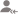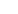true

Take Class 10 Tuition from the Best Tutors

•Affordable fees
•1-1 or Group class
•Flexible Timings
•Verified Tutors

Search in# Learn Mathematics with Free Lessons & Tips

Post a Lesson

All

All

Lessons

Discussion

Lesson Posted on 28/10/2022 Learn MathematicsVikas Mehra

I am an experienced ,qualified teacher with over 4 years of experience in teaching mathematics and economic....

Dislike Bookmark

Lesson Posted on 28/10/2022Vikas Mehra

I am an experienced ,qualified teacher with over 4 years of experience in teaching mathematics and economic....

Dislike Bookmark

Lesson Posted on 19/10/2022 Learn Mathematics +1Manjeet Khajuria

an = a+(n-1)d Where an=last term a=first term d=common difference n=number of term Note: last term is denoted by an or l Sn=n/2[2a+(n-1)d Sn=sum of all terms
Dislike Bookmark

Take Class 10 Tuition from the Best Tutors

•Affordable fees
•Flexible Timings
•Choose between 1-1 and Group class
•Verified Tutors

Lesson Posted on 19/08/2022Sheshan Kumar Jha

Hello Parents and Students,I have been teaching since I was in Class-9th as teaching has been my passion...

Dislike Bookmark

Lesson Posted on 04/08/2022 Learn Mathematics +1Anshuman Rai

I'm an ex government official with eight plus years of experience. Teaching is my passion and looking...

Dislike BookmarkRahul Kumar

Math, Science & Computer by an NITian tutor for Class X,IX with 15 years of experiecne

Hello Helen, This seems some issue with the network or app. You may call/write to customer support. Hope it helps.
Dislike Bookmark

Take Class 10 Tuition from the Best Tutors

•Affordable fees
•Flexible Timings
•Choose between 1-1 and Group class
•Verified Tutors

Lesson Posted on 16/06/2022 Learn MathematicsAshish K Sharma

I was a teacher and author and now, I am a Scientist. I have a PG degree in Mathematics as well a Diploma...

Q: The numerator of a fraction is 3 less than the denominator. If 2 is added to both the numerator and the denominator, then the sum of the new and the original fraction is 29/20. Find the original fraction. SOLUTION. Let the... read more

Q: The numerator of a fraction is 3 less than the denominator. If 2 is added to

both the numerator and the denominator, then the sum of the new and the

original fraction is 29/20. Find the original  fraction.

SOLUTION.

Let the numerator of the fraction be x. Then, according to the question,

Denominator = x + 3

Thus, the fraction is x  / (x+3). Now, adding 2 to both the Nr. And the Dr,

New fraction = (x+2) / (x+5).

Now, as per the question, we have,

[ x  / (x+3)] + [(x+2) / (x+5)] = 29 / 20. … Adding 2 to x and x+3

Simplifying, we have

( 2 * x^2 + 10 * x + 6) / ( x^2 + 8 * x + 15) = 29/20

On further simplification after cross multiplying, we have

11 * x^2 - 32 * x – 315 = 0

Factorising the above quadratic into 2 linear factors, we have

11 * x * (x – 7) + 45 * (x – 7) = 0

•                  11x + 45 = 0 or x -7 = 0

Now, the first root is -45/11 and the second root is 7.

Taking x = 7 as the numerator, we have denominator as 10.

Therefore our fraction is 7/10.

Note: We ignore the first root as it is negative and does not yield the satisfactory answer.

Dislike Bookmark

Lesson Posted on 09/06/2022 Learn MathematicsAshish K Sharma

I was a teacher and author and now, I am a Scientist. I have a PG degree in Mathematics as well a Diploma...

Q: Sum of the area of two squares is 400 square cm. If the difference of their perimeters is 16 cm, find the sides of the two squares. SOLUTION Let the side of the first square be ‘a’ and that of the second square be ‘b’.... read more

Q: Sum of the area of two squares is 400 square cm. If the difference of their perimeters is 16 cm, find the sides of the two squares.

SOLUTION

Let the side of the first square be ‘a’ and that of the second square be ‘b’.  then, according to the problem,

a^2 + b^2 = 400   …(1)

and                                  4a – 4b = 16          …(2)    Hint : Perimeter = 4 times the side of a square

Now, from (2), a = b + 4,

substituting this value of a in (1), we have

(4+b) ^2+b^2 = 400

Expanding the above we get the following quadratic equation:

b^2 + 4*b - 192 = 0             ….(3)

factoring the polynomial, we get,

b^2 + 16b-12b-192 = 0        …. (4)

simplifying, we obtain,

b(b+ 16)-12(b+ 16) = 0

• (b-12) (b+16) = 0

From which we get two solutions viz

b = 12 or b = -16

Now, since the side of a square cannot be negative, thus the correct solution is b = 12, whence, a = b + 4.

Thus, a = 16.

Therefore, the sides of the square are 12 and 16 cm respectively.

Dislike Bookmark

Lesson Posted on 08/06/2022Ashish K Sharma

I was a teacher and author and now, I am a Scientist. I have a PG degree in Mathematics as well a Diploma...

Arithmetic Progression Example. Q:If the sum of the first 7 terms of an AP is 49 and that of the first 17 terms is 289,find the sum of its first n terms. Solution: We know that the Sum... read more

Arithmetic Progression Example.

Q:If  the sum of the first 7 terms of an AP is 49 and that of the first 17 terms is 289,find the sum of its first n terms.

Solution:

We know that the Sum of the first n terms of an AP is given by:

Sn = (n / 2) * ((2 * a + (n-1) * d)).

Therefore, as per the first requirement,

49 = (7 / 2) * ((2 * a + (7-1) * d)).

• 98 = 14a + 42d
• 7 = a + 3 d                                    (1)

Similarly, as per the second requirement,

289 = (17 / 2) * ((2 * a + (17-1) * d)).

• 578 = 34a + 272d
• 17 = a + 8d (2)

Now, from (1), we have , a = 7 – 3d. Substituting this value of a in (2), we have

17 = 7-3d + 8d

Whence, 10 = 5d,

Thus, d = 2.

And, then a is 7 – (3 * 2) = 7 – 6 = 1

Thus, we have, a = 1 and d = 2. Now, the sum of the first n terms is

Sn = (n / 2) * ((2 * a + (n-1) * d)).

• Sn = (n / 2) * ((2 * 1 + (n-1) * 2)). ---- Substituting the values of a and d obtained earlier
• Sn = (n / 2) * ((2 + 2n -2))
• Sn = (n / 2) * (2n)
• Sn = n^2

Thus,

The sum of its first n terms = n^2.

Dislike Bookmark

Take Class 10 Tuition from the Best Tutors

•Affordable fees
•Flexible Timings
•Choose between 1-1 and Group class
•Verified Tutors

Lesson Posted on 02/06/2022Ashish K Sharma

I was a teacher and author and now, I am a Scientist. I have a PG degree in Mathematics as well a Diploma...

Q: Two casks A and B were filled with 2 kinds of sherry, mixed in the cask A in the ratio of 2:7, and in the cask B in the ratio of 1:5. What quantity must be taken from each to form a mixture which shall consist of 2 gallons of one kind and 9 gallons of the other, ... read more

Q:  Two casks A and B were filled with 2 kinds of sherry, mixed in the cask A in the ratio of 2:7, and in the cask B in the ratio of 1:5. What quantity must be taken from each to form a mixture which shall consist of 2 gallons of one kind and 9 gallons of the other,

SOLUTION.

Let us assume that we take ‘a’  gallons from the first cask and ‘b’ from the other cask. The, considering the first cask, we have :

2a/9 parts(or, units) of First type of sherry,S1,and

7a/9 parts(or, units) of First type of sherry,S2 .

And, considering the second cask, we have:

b/6 parts (or, units) of First type of sherry, S1,and

5b/6 parts (or, units) of First type of sherry, S2.

In the mixture, therefore, we have 2a/9+b/6 units of S1 and 7a/9+5b/6 units of S2.

Now, 2a/9+b/6 = (4a+3b) / 18 and 7a/9+5b/6 = (14a+15b) / 18

Therefore, as per the question, the ratio of S1 to S2 in the mixture is,

--    (  (4a+3b) / 18  )  /   ( (14a+15b) / 18 )     =  2 / 9

--   (4a+3b) / (14a+15b)  =  2 / 9

From which,

--        36a+27b = 28a+30b

--           8a = 3b

From which, we have finally,

a/b = 3/8

Thus, we have to take 3 gallons from cask A and 8 gallons from cask B to form the mixture.

Dislike Bookmark

UrbanPro.com helps you to connect with the best Class 10 Tuition in India. Post Your Requirement today and get connected.

Overview

Questions 1.5 k

Lessons 172

Total Shares117,504 Followers

## Top Contributors

Connect with Expert Tutors & Institutes for Mathematics

## Class 10 Tuition in:

x

X

### Looking for Class 10 Tuition Classes?

The best tutors for Class 10 Tuition Classes are on UrbanPro

• Select the best Tutor
• Book & Attend a Free Demo
• Pay and start Learning### Take Class 10 Tuition with the Best Tutors

The best Tutors for Class 10 Tuition Classes are on UrbanPro